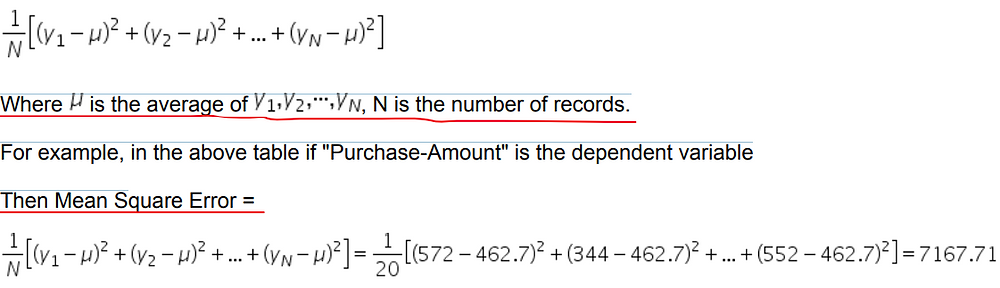Search

# What Is Decision Tree In Data Mining | Hire Data Mining Expert

### Decision Trees

Basic Concepts:

• Decisions trees generate models, represented by trees and rules

• Decisions trees are used for both classification (classification trees) and numeric prediction (regression trees) problems.

• Decision tree algorithms begin with the entire training dataset, utilize a splitting criteria to split the data into two or more subsets, and then repeatedly split each subset into smaller subsets until a stopping criterion is met. This process is called recursive partitioning.

• Strengths:

- Interpretability

- Ability to tackle "noisy" data

• Weakness:

- For large datasets, trees can be very complex

We use a customer dataset to illustrate a few terms:Independent variables and Dependent Variables

For business operations, it is very important to predict how much a customer will purchase and who are our best customers. For example we will use variables “Age”, “Year-of-Education” and “Income” to predict variable “Favorite”. In this case, predictors are “Age”, “Year-of-Education” and “Income”, the variable to be predicted is the variable “Favorite” which is a categorical variable. So it is a Classification prediction. We also call “Age”, “Year-of-Education” and “Income” Independent Variables. We call “Favorite” Dependent Variable . if we use variables “Age”, “Year-of-Education” and “Income” to predict variable “Purchase-Amount”, then “Purchase-Amount” is dependent variable. Since “Purchase-Amount” is numerical, this is a regression prediction.

Splitting

Dataset is split using an Independent variable.

For example, the above dataset can be split into 2 sub-datasets using independent variable “Age”. If the rule for the first sub-dataset is “Age is less than or equal 22.5”, then it has 2 records (customers with ID=10 or 14). And the rule for the second sub-dataset is “Age is greater than 22.5”, which contains the remaining 18 records.

Impurity Measurement

Impurity is measured using Dependent Variable. For a categorical dependent variable, we use Gini to measure its impurity. For a numerical dependent variable, we use "Mean Square Error" to measure its impurity. There are other impurity measurements, which will not be covered by this course.

Gini Calculation

We now describe how Gini is calculated.Mean Square Error Calculation

Mean Square Error for numerical dependent variable isExample

If we use "Age", "Year-of-Education" and "Income" to predict "Favorite", then the above dataset decision tree result is shown hereFor this tree, each rectangle represents a tree node. a terminal node is a leaf

The top (root) node shows

Age ≤ 22.5

gini = 0.48

samples = 20

value = [8, 12]

class = YES

The line ”value=[8 12]” means the dataset has 8 NOs and 12 YESes of variable “Favorite”. The line “samples=20” means the number of records is 20. Using gini formulae, we get gini=The left node of the second row shows

gini = 0.0

samples = 2

value = [2, 0]

class = NO

Decision Tree Algorithms

• A node represents a set of data

• The entire dataset is represented as a root node

• When a split is made, several child nodes are formed

• If a node is not to be split any further, it is called a leaf (or terminal node); otherwise, it is an internal node (or non-terminal node).

• In a binary tree which we use, each split produces exactly two child nodes

• A node is split according to Decision Tree Splitting criterion, which is that we exhaust all the possible splits (by selecting different independent variable and using different cutting value e.g Age, 22.5 ) so that the Impurity (measured by Gini for categorical variable or Mean Square Error for numerical variable) Decrease of the selected split is maximal.

The Issue Of Tree Parameter

It is always possible to construct a sufficiently large tree to drive the training error rate down to zero. For example, a tree having one record on each of its leaves has zero error, but this tree overfits the training data and is not likely to work well when used for unseen data. If the dataset is divided into two sets: training (e.g., 80%) and validation (e.f., 20%) sets. We can tune decision trees by building the decision trees based on the training set and find the best tree performance using the validation test set. In general, you can observe tree performance changes by changing Minimal Impurity Decrease .

Minimal Impurity Decrease is the most important tuning parameter for decision tree, it requires the Impurity Decrease of any split must be above this value. We can change Minimal Impurity Decrease to tune our Decision Tree so that it performs best on the test set.

Basically we need to try a few times using different Minimal Impurity Decrease values to find the best value.